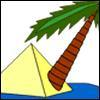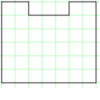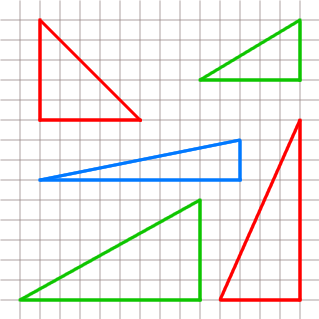#### You may also like### Triangle Island

You have pitched your tent (the red triangle) on an island. Can you move it to the position shown by the purple triangle making sure you obey the rules?### Triangle Transformation

Start with a triangle. Can you cut it up to make a rectangle?### Rod Area

This task challenges you to create symmetrical U shapes out of rods and find their areas.

# Uncanny Triangles

## Uncanny Triangles

Thomas, Jane and Anna were drawing right angled triangles on squared paper. Their triangles had two sides which were an exact number of squares long and could not be longer than $15$ squares. These are Jane's triangles:They were calculating the areas of the triangles.

"I've got one triangle where the area and the sum of the lengths of the two shorter sides come to exactly the same number!" exclaimed Anna, "Look, it's that one!"

Thomas looked at his work. "How uncanny - but so have I! But look at it. It's quite a different shape from yours."

What were the measurements of the triangles they had drawn?

### Why do this problem?

This problem needs a systematic approach. It is useful when learners are studying the area of right-angled triangles. It is important to remember that it is only the two shorter sides of the triangles that are being considered in the problem, not all three sides.

### Possible approach

Most learners will need to draw some right-angled triangles on squared paper to get a feel for the problem.

### Key questions

Where is a good place to start?
Have you looked at some of the examples given in the problem?
Do you need to draw all possible triangles to see if any meet the requirements?
If you double the length of the sides how does the area change?

### Possible extension

Learners could consider fractional measures or look for triangles where the sum of the three sides is numerically equal to the area.

### Possible support

Suggest drawing some right-angled triangles on squared paper and counting the squares to find the area.